Basics of Geometry
edHelper subscribers - Create a new printable

Number of Keys
 Select the number of different printables: 1 key 2 keys 3 keys 4 keys 5 keys

Number of Pages (for each key)
 Select the number of pages: 1 page 2 pages 3 pages 4 pages

Include an answer key (answer keys will be at the end of the printable)
High School Geometry
High School Geometry
Math

 Name _____________________________Date ___________________ (Key 1 - Answer ID # 0916344)
1.   The acute angles of a right angle are ___________.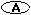scalene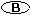isosceles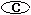obtuse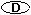supplementary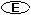complementary
2.   A triangle with three acute angles with equivalent measure is ___________ triangle.an acutea rightan obtusean equiangular
3.   The three angles in triangle PFL are in the ratio of 8:8:29. What is the measure of each angle?
4.   A scalene triangle has ___________.no congruent sidesonly two congruent sidesall congruent sides
5.   The measure of an exterior angle of a triangle ___________ the sum of the measures of the two nonadjacent interior angles.is greater thanis not related tois equal tois less than
6.   A triangle is the figure formed by the union of three segments determined by ___________.three skew pointsany three pointsthree collinear pointsthree noncollinear pointsthree angles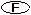three rays
7.   ___________ of a triangle is the angle adjacent to an interior angle of a triangle that is formed when one of the sides of the triangle is extended.An interior angleAn isoscelesA complementary angleA remote interior angleAn exterior angle
8.   What is the sum of the measures of the angles in a triangle?4590205360180None of the above

Basics of Geometry
edHelper subscribers - Create a new printable

Number of Keys
 Select the number of different printables: 1 key 2 keys 3 keys 4 keys 5 keys

Number of Pages (for each key)
 Select the number of pages: 1 page 2 pages 3 pages 4 pages